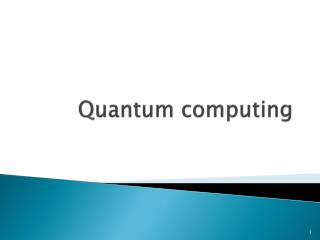DownloadDownload PresentationQuantum computing

# Quantum computing

Télécharger la présentation## Quantum computing

- - - - - - - - - - - - - - - - - - - - - - - - - - - E N D - - - - - - - - - - - - - - - - - - - - - - - - - - -
##### Presentation Transcript

1. Quantum computing

2. Motivation • Two approaches to increase efficiency: • Nanotechnologies • Quantum computers • Take advantage of quantum effects and design quantum algorithms. • Reversibility

3. Content • Qubits • Multiple qubits • Computational basis • Quantum gates • Bell states circuit • Teleportation circuit

4. Qubits • The equivalent to a classical bit • Can be in the states or as classical bits • But also in a superposition state • Possibility of representing different numbers at the same time with only one qubit • But measurement put it back in the state or with probabilities and

5. Multiple qubits • 4 computational basis states for a 2 qubit system • Superposition state

6. Computational basis

7. Quantum NOT gate

8. Hadamard gate • After measurement becomes or

9. CNOT gate

10. Toffoli gate

11. Bell states circuit

12. Quantum teleportation

13. Quantum teleportation

14. Quantum teleportation

15. Bibliography • M.A. Nielsen & I.L. Chuang, Quantum Computation and Quantum Information, Cambridge University Press, 2000. • P. Kaye, R. Laflamme & M. Mosca, An Introduction to Quantum Computing, Oxford University Press, 2007. • M. Nakahara & T. Ohmi, Quantum Computing: from Linear Algebra to Physical Realizations, CRC Press, 2008. • T. Hey, Quantum Computing: an introduction, Computing & Control Engineering Journal, vol. 10, n°3, 105-112, 1999. • A. Barenco & al., Elementary gates for quantum computation, Phys. Rev., A52, 3457-3467, 1995. • D. Deutsch, R. Jozsa, Rapid Solution of Problems by Quantum Computation, Proceedings: Mathematical and Physical sciences, vol. 439, n°1907, 553-558, 1992. • P. Shor, Algorithms for Quantum Computation: Discrete Logarithms and Factoring, Proceedings: 35th Annual Symposium on Fundamentals of Comp. Science (FOCS), 124-134, 1994.